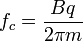Home » Posts tagged 'revolution'

# Tag Archives: revolution

## Size of body neglected in Circular motionWhy in  circular  motion the  size  of  the moving body is  neglected?

Answer:

Not only in circular motion, but in entire kinematics we discuss the case of point objects only. In kinematics we study motion only and not the objects undergoing the motion.

A point object is an object having negligible dimensions compared to the distance travelled by it. For example, a car moving from Delhi to Mumbai can be considered a point object.

Further, when we discuss the concepts in Kinematics, even a large object is dealt considering the entire body to be a single point. This point will be the centre of mass of the body.

If a person is revolving a stone tied to a string, the centre of mass of the stone is taken for determining the length starting from the point about which it revolves.

In dynamics, we have to consider the shape and size of the body also.

## Why electrons cannot be accelerated using a cyclotron?

In a cyclotron, the most important condition is that of the cyclotron frequency. The frequency of the square wave oscillator connected to the dees of the cyclotron must match the frequency of revolution of the charged particle being accelerated.

For ordinary ions, once the  frequency is set there is no need to change or adjust the frequency.

The equation for cyclotron frequency isAs it is clear from the above equation that the cyclotron frequency is inversely proportional to mass of the ion. The frequency of revolution is apparently constant for ordinary ions.

If an electron is accelerated in a cyclotron, it quickly picks up high-speed comparable to the speed of light because of its light mass. The speed comparable to the speed of light is called relativistic speed. At relativistic speeds, mass is not constant but varies according to the relation.As per the equation as speed increases, relativistic mass increases. This will change the frequency of revolution and the revolution will go out of phase. The acceleration will stop.

## Why a cyclotron cannot be used to accelerate an electron?

UTKARSH AGRAWAL asked:

why a cyclotron cannot be used to accelerate an electron?

Answer:

The mass of electron is very less. So, when it is accelerated in a cyclotron, its speed increases rapidly and reaches relativistic values. (i.e. speeds comparable to the speed of light) Then the mass of the electron increases according to the equation,

This change in mass changes the frequency of revolution of electron. SinceTherefore the electron goes out of phase with the cyclotron frequency and acceleration stops.

This happens  because as the relativistic mass increases due to increase in speed, the frequency decreases which means that the time period of revolution increases. Therefore the polarity of the Dees reverses before the electron completes half revolution and the accelerator will not function properly.

### Visitors So Far @ AskPhysics

• 2,115,374 hits

### Subscribe to Blog via Email

Enter your email address to subscribe to this blog and receive notifications of new posts by email.

Join 4,004 other subscribers

### askphysics@TwitterAsk Physics
@askphysics